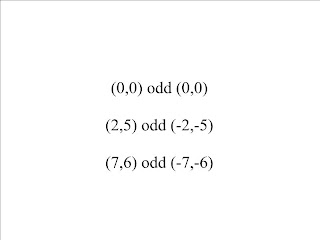## Monday, March 3, 2008

### Odd, Even, and Inverses

Hi its me benofschool and here is today's scribe. Let's begin.

We started off with reviewing what an odd and even function was. An even function is a function that is symmetrical across the y-axis as explained in the 2nd slide of the slide show. An odd function is a function that is symmetrical about the origin. For example if a coordinate found on a function is (2,3) then the odd coordinate of the odd function would be the opposite sign for the x and y coordinate which would be (-2,-3).Next we learned to find out if a function is even, odd, or neither. To find the even function, take the given function and make f(x) into f(-x). Look at the first question on the 4th slide of the slide titled March 4. To see if the function was even make it f(-x) which makes all x values negative. Then simplify. If the result was the same as the original function then it is even.

For an odd function start off the same way as the even function procedure and if it is not equal to the original function then make f(x) into -f(x). That means put the original function in brackets and multiply it by negative 1. Then if it is equal to f(-x) it is odd. But if it was not equal to it then it is neither.

In symbolic format for an even function: f(x)=f(-x). For an odd function: f(-x)==f(x).

For the 5th slide we had to find the odd of the function in the 1st quadrant. To find it we had to get the main points on the graph and find the odd equivalents of them:Now we began inverse functions. One anecdote would be a baby invading a clean room makes a messy room. That would be the original function. An inverse function would be a function that undoes what the original function did so the inverse of the baby play would be the parents go into the messy room and makes it clean again. You can see it visually in the 7th slide.

In mathematical talk. The Inverse of a function undoes what the original does. The domain of a function is the range of the inverse while the range of the function is the domain of the inverse function. Symbolically: (a,b) ---> (b,a).

If you were wondering why I was stating different things in different ways like symbolically and graphically which are all the same block of wood. A block of wood is Mr.K's favourite teaching tool. It shows that a function or a graph can be expressed in 3 different ways: graphically, symbolically, numerically; in some cases verbally.

Back to the class. We were then told to find the inverse of a function. If a function was y=f(x) then to find the inverse we switch the variables: x=f(y). But we, smarter than the average bear (Mr.K's famous sayings), must solve for y like in the 8th slide. y=f(x) ---> x=f(y).

We can then look at it conceptually (same block of wood) which is found of the 8th slide on the T-chart. We showed what x went through to equal y so the inverse does the opposite in the reverse direction shown on the slide.

Okay lets graphicall look at all of this madness. Lets take a look at the final slide. The graph of f(x)=x^3. The axis of symmetry is y=x. so if we could fold the graph along the axis of symmetry it would also become the inverse. Or if we switched the x values with y values and vice versa we get the inverse function.

That was all for today and unfortunately it was only one period long so we couldn't complete that last question on the board so hopefully that will be tomorrow. So homework is the exercise #9 and to find a site that has to do with circular functions and Delicious it with the tags: pc40sw08, the unit, and preferably your blog name.

The next scribe will be Hi I'm Justus.

Bai Bai